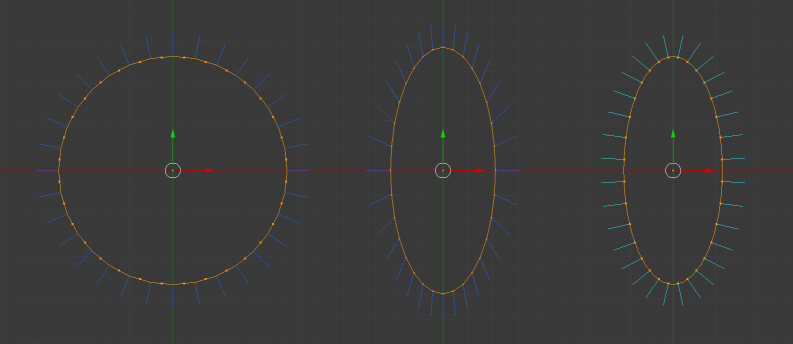# Baking Transformations

## Baking

Usually, within Babylon.js, positioning, rotating and scaling a mesh changes its world matrix only and the vertex position data of a mesh is left unchanged. In certain situations you might be interested in applying a transform (position, rotation, scale) directly to the mesh vertices and leave world matrix unchanged. This is called baking and, of course, changes the center of transformation of the mesh, and can be useful in the following situations:

• building a set of static geometry
• randomizing a series of mesh copies
• mirroring a mesh along an axis
• etc.

The most straight forward way is to apply a transformation to the mesh. For example take a box of side 1 and position it at (0, 3, 0). Its vertices are stored as (-0.5, -0.5, -0.5), (0.5, -0.5, -0.5), (0.5, 0.5, -0.5), (-0.5, 0.5, -0.5), (-0.5, -0.5, 0.5), (0.5, -0.5, 0.5), (0.5, 0.5, 0.5), (-0.5, 0.5, 0.5) with its local origin of (0, 3, 0) stored in the world matrix. When this current transformation is baked into its vertices, the vertices are now stored as (-0.5, 2.5, -0.5), (0.5, 2.5, -0.5), (0.5, 3.5, -0.5), (-0.5, 3.5, -0.5), (-0.5, 2.5, 0.5), (0.5, 2.5, 0.5), (0.5, 3.5, 0.5), (-0.5, 3.5, 0.5) with a local origin of (0, 0, 0) stored in the world matrix. Any rotation now takes place with the center of rotation 3 below the middle of the box.

Baking a current transformation: Baking Current Transformation

When the current transformation is baked into the mesh the local origin of the mesh is also altered so that the location and orientation of the mesh within the seen is not changed.

There is also a method, bakeTransformIntoVertices, to bake a matrix as a transformation into the vertices. This will bake the transformation provided by the matrix directly into the mesh vertices changing their values but leaving the world matrix unchanged.

usage:

mesh.bakeTransformIntoVertices(matrix);

Baking using matrices Baking Using Matrices

## Use With Scaling

When baking scaling the normals are simply scaled in their current direction and so baking a scale can often give unrealistic results for lighting. To correct this normals need to be recomputed. This is illustrated in the following picture:In the above picture, you can see an untransformed mesh on the left, the same mesh with a baked scaling along the X axis in the middle and on the right, the mesh with its normals correctly recomputed.

You can do a recomputation of your normals like so:

var indices = mesh.getIndices();var normals = mesh.getVerticesData(VertexBuffer.NormalKind);BABYLON.VertexData.ComputeNormals(positions, indices, normals);mesh.updateVerticesData(VertexBuffer.NormalKind, normals, false, false);

Note: recomputing the normals of your mesh may not be an ideal solution, as the results may be wrong in some parts of the mesh (e.g. seams on a sphere).

Unless you have good reasons to use baking transformations then your are better with parents and pivots.